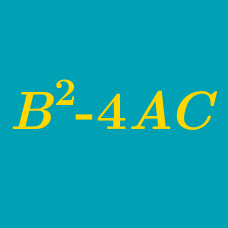Algebra

# Finding the Discriminant of a Quadratic

What is the discriminant of the quadratic polynomial $$4x^2+11x+1$$?

If the discriminant of the quadratic $$2x^2 + ax + 72$$ is 0, what is $$a$$ if $$a > 0$$?

If $$a$$ is a nonzero real number, what is the discriminant of the quadratic polynomial $ax^2-8x-\frac{1}{a}?$

If $$a$$ and $$b$$ are real numbers such that the discriminant of the quadratic polynomial $\frac{11}{2}x^2+2(a+11b)x+8ab$ equals $$0$$, what is $$\frac{a}{b}$$?

What is the discriminant of the quadratic $$x^2 - 10x + 2$$ ?

×